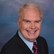# ‘Math Solutions' For Wastewater Operator CertificationBy Daniel Theobald, "Wastewater Dan"The introduction to Water Online’s new “Math Solutions” series, presented by wastewater consultant and trainer Dan Theobald (“Wastewater Dan”), instructs operators on six essential calculations.

The thought process behind a regular presentation of “Math Solutions” comes from my 24-plus years of training experience in wastewater. I learned that many operators were held back from advancing their operator license because they struggled with the mathematics portion of wastewater operator certification examinations.

I developed a tool for operators – represented through video tutorials – offering mathematical templates for performing accurate wastewater calculations. The tool also provides definitions, explanations, and alternative applications for the calculations beyond the specific examples presented. In addition to operators, it may also serve educators, engineers, regulators, and others.

Practical calculation applications for this tool include volume, flow rate conversions, poundage, and more.

Practically applying volume includes capacity calculations of pipes, tanks reservoirs, etc. Flow rate conversions are used applying time to calculated volumes. Poundage calculations utilize flow rate conversions and volume calculations to quantify the amount of pollutants.

Volume Calculations

Calculate gallons of circular tank:

Calculate gallons in treatment tank:

Flow Rate Conversions

Convert MGD flow to GPM:

Convert flow to minutes from hours:

Poundage Calculations

Calculate lbs. using flow MGD:

Calculate lbs. of lime to stabilize sludge:

This is the first presentation in my series of “Math Solutions.” If you have specific wastewater math queries, please submit a question.